### Home > MC2 > Chapter 3 > Lesson 3.1.3 > Problem3-29

3-29.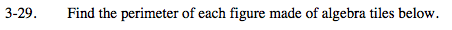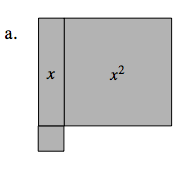Refer to the solutions for 3-20 or the Math Notes box of Lesson 3.1.3.

Remember, the tiny square represents a unit piece, which is a 1 × 1 square.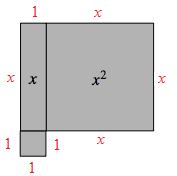4x + 4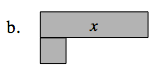Refer to the solutions for 3-20 or the Math Notes box of Lesson 3.1.3.

Since part of the side with length x touches the unit square, the side that is included in the calculation of the perimeter is x −1.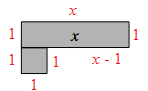Do you understand why the length of one side is labeled
x −1?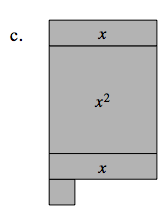Refer to the solutions for 3-20 or the Math Notes box of Lesson 3.1.3.